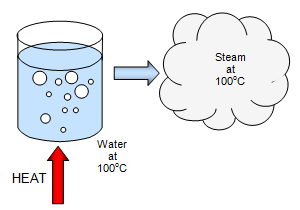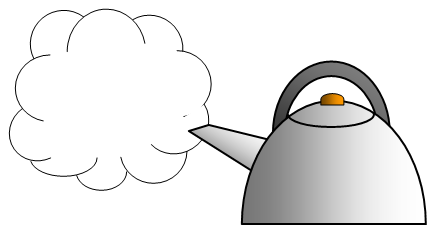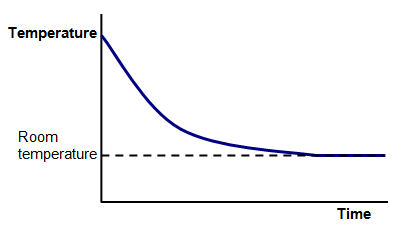# Latent heatWhen a substance changes its state from a solid to liquid or from a liquid to a gas heat energy is needed. This energy is used not to heat up the substance but to separate the molecules from each other.

This energy is called LATENT HEAT energy.
While a solid is melting and while a liquid is boiling there is no temperature change. The temperature only changes when the change of state is complete.

The amount of heat needed to change the state of 1 kg of a substance is called the SPECIFIC LATENT HEAT of the substance.

The units for specific latent heat are joules/kilogram (J/kg).

It may take a different amount of heat to change the solid into a liquid than to change the liquid into a gas and so a substance will have two specific latent heats.

The specific latent heat of fusion - the heat needed to turn 1 kg of solid into a liquid at its melting point.
The specific latent heat of vaporisation - the heat needed to turn 1 kg of liquid into a gas at its boiling point.

The heat needed is given by the equation:

Heat energy to melt or boil something = mass x specific latent heat

Example problems
1. Find the energy needed to change 4 kg of water at 100oC into steam at 100oC.
Specific latent heat of vaporisation of water = 2 260 000 J/kg.

Energy required = 4 x 2 260 000 = 9 040 000 J.
2. Find the energy needed to change 500 g of ice at 0oC to water at 0oC. Specific latent heat of fusion of water = 334 000 J/kg.

Energy required = 0.5 x 334 000 = 167 000 J.
Much more heat is needed to turn 1 kg of water into steam than to melt 1 kg of ice.

When a gas condenses and when a liquid freezes latent heat is given out. The amount of heat that was used to melt or boil the substance is released. When steam at 100oC condenses to water it gives out a lot of heat and we can use this fact to explain why a scald from steam at 100oC is much more painful than a scald from water at the same temperature.

Example problems
Scalds from water and steam
We assume that our hand is at 37oC, and when we put 100 g of water at 100oC on our hand the water will cool to 37oC. The heat energy "lost" by the water will be gained by our hand.

Heat "lost" 0.1 x 4200 x 63 =26 460 J.
But if the 100 g had been steam then the steam would first have to condense.
Heat "lost" in condensing = 0.1 x 2260000 = 226 000 J plus a further 26460 J in cooling, a total of 252 460 J.

This is nearly ten times as much as the water alonel

We can also calculate the mass of water that will be turned to steam if a kettle is left boiling (at 100oC) for 5 minutes. Will the kettle boil dry?

Example problemsPower of kettle = 2 kW
Time of boiling = 5 mins = 600s
Heat energy produced in that time = 2000 x 600 = 1 200 000J
Heat energy needed to turn 1 kg of water into steam = 2 260 000 J
Mass of water turned to steam = 1 200 000/2 260 000 = 0.53 kg = 530 g.

The kettle will usually have more water in it than this at the start and so it should not boil dry. We have assumed that there is no heat lost by the kettle.

The large specific heat and specific latent heat of water make it a very good liquid for use as a coolant in many types of machinery. It is also available very cheaply and in large quantity.

## CoolingAn object gets colder because it is loosing heat energy to its surroundings. As it cools its temperature obviously drops. This goes on until the object reaches the same temperature as its surroundings. You can tell when this happens because the graph of temperature against time becomes flat.

Another reason for the graph flattening out is that the substance might be turning from a gas into a liquid or from a liquid into a solid.

A VERSION IN WORD IS AVAILABLE ON THE SCHOOLPHYSICS USB